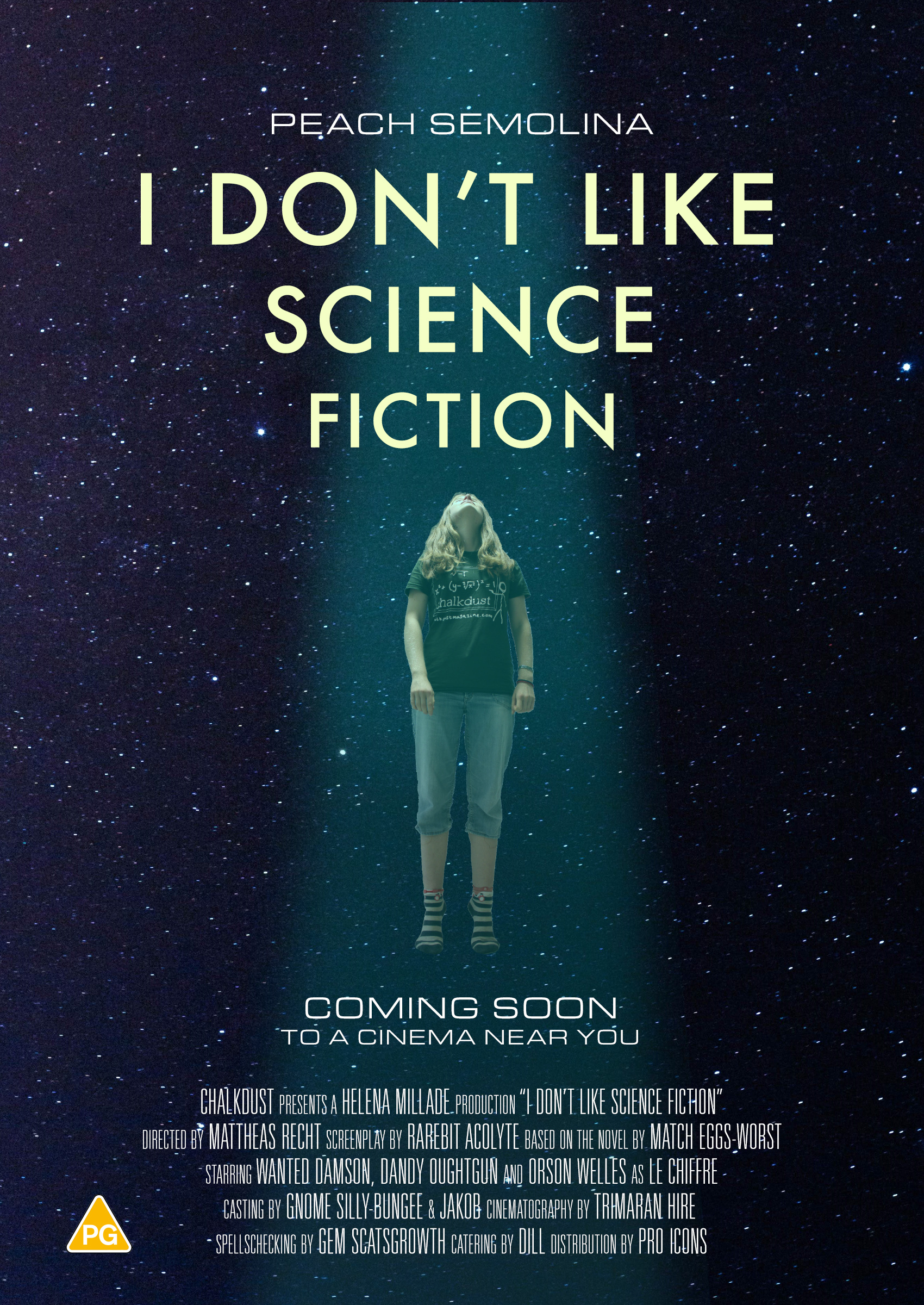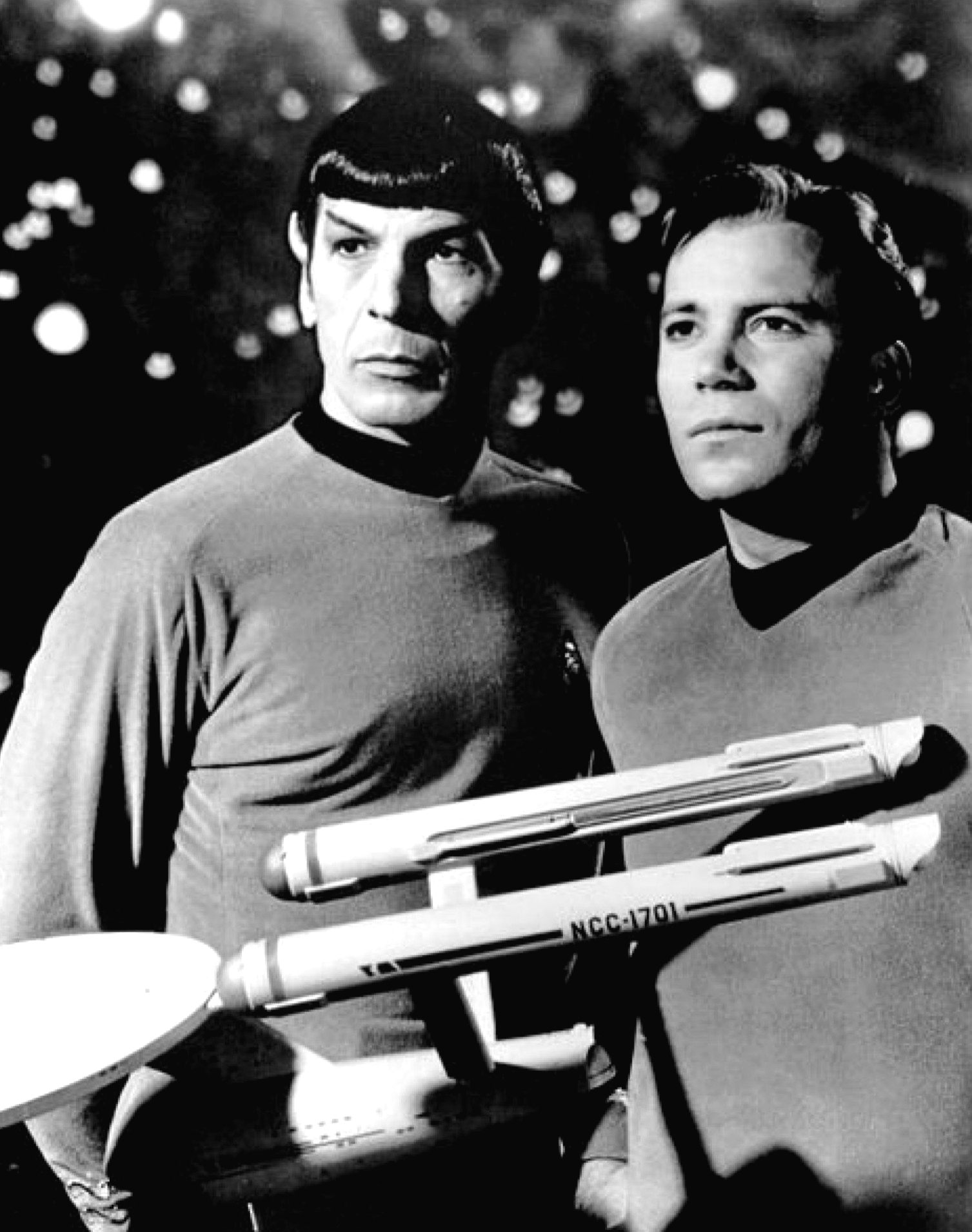# I don’t like science fiction

Peach Semolina admits her true feelings about science fiction, and delves into the maths of quantum teleportation.I have a confession. I’m quite nervous to say this really, but it’s an inescapable fact and I feel the readers of Chalkdust need to know that people like me exist. Here goes…

I don’t like science fiction.

Phew. That’s a huge weight off my shoulders. It’s true though. Despite being a proud nerd, I just can’t get into sci-fi. It’s not that I don’t like fiction (I do) and it’s certainly not that I don’t like science, but together it does nothing for me. And I know I’m missing out on a lot. I will never know that Darth Vader is Luke’s father. Oops. And I will never know how a flux capacitor works. But one thing I don’t need to miss out on is learning about teleportation. Specifically quantum teleportation. Because quantum teleportation exists at the very least in the mathematical world, even if not yet in the physical world.

## Quantum plumbing

Even the least discerning among you will have worked out by now that this article is about quantum teleportation. And I promise that I will get onto that soon (I’m familiar with Chekhov’s gun). But first, there is a bit of groundwork that needs doing. I’m going to assume that the average reader has no knowledge of quantum mechanics, so we must start at the beginning. What on earth is it?!

At its core, quantum mechanics is the study of very small things, usually subatomic particles and other things on a subatomic scale. I’m sure that’s not as exciting as you were expecting. Unfortunately, marketing and PR experts have got their hands on the word ‘quantum’ and warped it beyond recognition. But part of the incredible nature of quantum mechanics is how real and everyday it is, and yet it has so many ethereal and otherworldly consequences.

A quick side note on nomenclature (though I’m sure an expert would disagree with me): ‘quantum physics’ and ‘quantum mechanics’ are terms which are largely used interchangeably, at least at the level on which we’ll be discussing the subject. Quantum decorators, quantum plumbers, and quantum interior designers alas don’t exist. Yet.

The first idea introduced in most quantum mechanics courses is the concept of quantisation of energy. Put simply, it means that particles can only absorb energy (as radiation) in specific packets, named quanta. Hence the name.

Another key tenet of quantum mechanics is the idea of wave–particle duality. This asserts that every quantum entity can be treated as either a wave or as a particle, and these two types of object are equivalent. But these two concepts are not relevant for teleportation. The key principle we need is the uncertainty principle.

## Schrödinger’s ET

The uncertainty principle has broken into the realms of popular science via the famous thought experiment: Schrödinger’s cat. In this, you are asked to imagine a box in which there is a cat, some radioactive material, and acid. The box is set up with equipment so that if the radioactive material decays, the acid is released and the cat dies. The uncertainty comes in because radioactive decay is a random event and so, without opening the box, the cat could be either dead or alive and only by opening the box do you learn which.Schrödinger’s cat isn’t happy about being forced into a box with some acid and radioactive material.

I can’t go any further without asking you all to please make sure this remains a thought experiment as I strongly oppose animal cruelty. Do it with an alien or something instead. (Does it count as animal cruelty? Am I going to get sued for this? Do aliens actually exist?)

Schrödinger’s thought experiment suggests that quantum mechanics works in much a similar way to the cat in the box and, up to a point, it does. Quantum entities can be in one of a discrete set of states just as the cat can be dead or alive. It’s also true that until you measure the quantum entity, you don’t know which state it’s in, just as you don’t know whether the cat is dead or alive until you open the box. But this is where the model starts breaking: the cool thing about quantum mechanics is that the reason you don’t know what state an entity is in until you measure it is that, unlike the cat, it isn’t in any state until you measure it.

This takes a bit of time to wrap your head around so I’ll explain it in a bit more detail. Up until you measure a quantum entity, it exists in a superposition (ie a combination) of all possible states. When measured, the entity collapses into a given state and remains in that state from then on. There are set probabilities of it collapsing into each state on measurement and this is dependent on the composition of the superposition of the states.

This is objectively awesome.

## Quantum of solace

What does this look like in practice? A single bit of quantum information (a qubit) is denoted by the quantum state $\left| \psi\right\rangle$. I’m going to mainly deal with two dimensions here because we don’t need any more for the scope of this article, but it is possible to consider more than two. Let’s consider an two-dimensional orthogonal basis: $\left\{\left|0\right\rangle, \left|1\right\rangle \right\}$. Don’t worry if this seems scary, hopefully it’ll become clear in good time.

Those of you familiar with linear algebra will have heard the term ‘orthogonal basis’ before. In linear algebra, vectors are orthogonal if they are perpendicular to each other. A basis is a set of vectors which can be added together in different combinations to form any other vector. An orthogonal basis is therefore a set of perpendicular vectors from which any other vector can be formed, and as a side effect of the orthogonality, this can be done in precisely one way.

The definition of an orthogonal basis in quantum mechanics is just the same. $\left|0\right\rangle$ and $\left|1\right\rangle$ are two quantum states which can be added to form any other quantum state in a unique way. This isn’t where the parallels between quantum mechanics and linear algebra end though. In fact, qubits can be described as vectors. So that is what we shall do.

We can represent our basis as $\left|0\right\rangle = \begin{pmatrix} 1 \\ 0 \end{pmatrix}, \left|1\right\rangle = \begin{pmatrix} 0 \\ 1 \end{pmatrix}.$ This means that a general qubit can be written $\left| \psi\right\rangle = a\left|0\right\rangle + b\left|1\right\rangle = \begin{pmatrix} a \\ b \end{pmatrix},$ where $a$ and $b$ are real numbers. Quantum states are normalised, so in two dimensions, this means that ${a}^{2} + {b}^{2} = 1$. One final bit of notation here (it’s a surprise tool that will help us later). We can define $\left\langle\phi\right|$ as: $\left\langle\phi\right| = {\left|\phi\right\rangle}^{\dagger} = a^* \left\langle0\right| + b^* \left\langle1\right| = \begin{pmatrix} a^* & b^* \end{pmatrix},$ where $a^*$ is the complex conjugate of $a$. Linear algebra fans will recognise that $^\dagger$ is the adjoint of a vector. Linear algebra haters need not worry—the word ‘adjoint’ will never be spoken of again (in this article). The above is a general linear algebra result, but for quantum states specifically, $a$ and $b$ are real so we can simplify this to $\left\langle\phi\right| = \begin{pmatrix} a & b \end{pmatrix}$ .

## Lights, camera, action!

Now we have our qubits, we can do things with them! We generally refer to ‘things we do to qubits’ as ‘actions’ (apparently ‘things we do to qubits’ isn’t snappy enough). Don’t ask me how these actions are carried out. Just trust me that they are.It screened in a living room in Bradford whilst Sundance was underway; it was mentioned in the same sentence as the Palme d’Or; it was described as ‘watchable’ by Chalkdust reviews. Coming soon to a cinema near you.

Some of these actions can be represented by matrices, and to find the effect of the action on a qubit, we simply multiply the vector representing the qubit by this matrix. Do you want some examples? Of course you want some examples. Here are a few of my favourites:

• First up, we have an action which I shall refer to as $X$. $X$ swaps the $\left|0\right\rangle$ and $\left|1\right\rangle$ components and is represented by the matrix $\begin{pmatrix} 0 & 1 \\ 1 & 0 \end{pmatrix}.$
• The second action keeps the $\left|0\right\rangle$ component as is, but flips the sign of the $\left|1\right\rangle$ component. I shall call this action $Z$, because this is how it is generally known. $Z$ is represented by the matrix $\begin{pmatrix} 1 & 0 \\ 0 & -1 \end{pmatrix}.$
• The final action I want to share with you is so special that it is named after a person: the Hadamard gate. It’s not a coincidence, by the way, that the use of the word ‘gate’ gives logic and computing vibes. I’ll let you think about why that might be for yourself. The Hadamard gate, $H$, is defined by the (normalised) matrix $\frac{1}{\sqrt{2}} \begin{pmatrix} 1 & 1\\ 1 & -1 \end{pmatrix}.$ Graphically, this is actually a reflection in the mirror line at an angle of $\pi/8$ to the $x$-axis.

## A state of collapse

As I mentioned before, we can work out the probability that the qubit (say $\psi$) is in a certain state (say $\phi$) when measured. To do this, we take the inner product: $\left\langle\phi \middle| \psi\right\rangle$ (I told you that notation would be useful!) and that equals the probability. A quick demonstration, for those who are new to this:

$\begin{array}{rr}\left|\psi\right\rangle = a\left|0\right\rangle + b\left|1\right\rangle \\ \left|\phi\right\rangle = c\left|0\right\rangle + d\left|1\right\rangle\end{array} \implies \; \left\langle\phi \middle|\psi\right\rangle = \begin{pmatrix} c & d \end{pmatrix} \begin{pmatrix} a \\ b \end{pmatrix} = ca + db.$

Because of our normalisation, this is a real number less than or equal to 1.

Once we’ve conducted this measurement (just like with the actions, please don’t ask me how that happens in practice), the qubit collapses into whichever state the result of the measurement is. If it makes more sense to you, you can think of this as it becoming a qubit that, when measured, gives the same result as the initial measurement with probability 1. If that makes less sense to you, then forget I said it.

## Around the world in eighty milliseconds?

There we have it! Teleportation! Allons-y! Geronimo!(In James Doohan’s voice:) “Captain, I cannae get ethical approval for beaming you up.”

OK, so I’ll admit that knowing this is all very well but I’ve not told you how to actually do this in reality, which is largely because I don’t actually know. Plus even if I had explained how to do this in practice, at best, you could teleport quantum entities one by one. I’m sure some of you were hoping to learn how to teleport, say, animals but that brings in all sorts of ethical and philosophical quandaries on top of the practicalities.

I started by saying how I wasn’t missing out on science fiction because I had mathematics, and I won’t ask whether or not you agree. I know the audience I’m talking to. Sci-fi nerds among you may in fact feel victorious. Perhaps you would point out that at least in science fiction it is possible to teleport people, and that if I want to truly experience teleportation, I have no choice but to engage with sci-fi. But to that, I have one thing to say. That sounds excruciatingly painful. And teleporting sounds pretty uncomfortable too.To protect the author from the science fiction community, we have given them the pseudonym Peach Semolina are are not publishing Sophie Maclean’s real name. Ah. Well, at least we didn’t give away her Twitter handle.